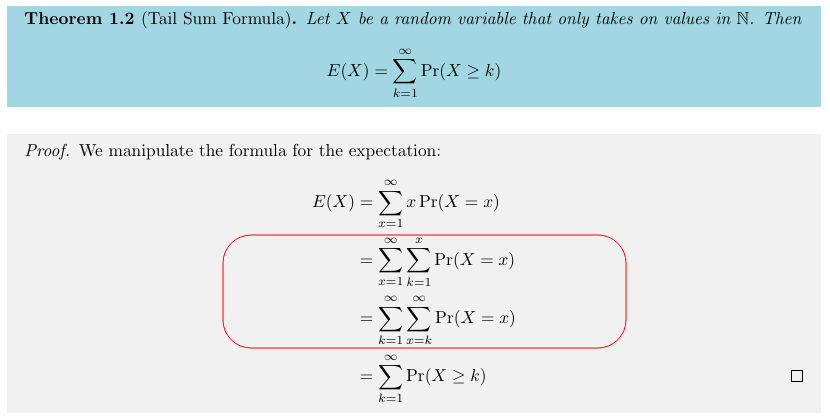$E(X) = \sum_{x=1}^{\infty} P(X \geq x)$

$E(X) = \sum_{x=0}^{\infty} P(X \gt x)$

公式证明

Sinho Chewi 的笔记 上，可以看到这一公式的证明。$E(X) = \sum_{x=1}^{\infty} \sum_{k=1}^{k=x} P(X=x)$

$Row(X=x) = \sum_{k=1}^{k=x} P(X=x)$ $E(X) = \sum_{x=1}^{\infty} Row(X=x)$$E(X) = \sum_{k=1}^{\infty} Column(K=k)$ $Column(K=k) = \sum_{x=k}^{\infty} P(X=x)$

$E(X) = \sum_{k=1}^{\infty} \sum_{x=k}^{\infty} P(X=x)$

公式应用

Probability for Statistics and Machine Learning 一书中，对此定理有一个有趣的例题：

$P(X > n) = p^n + (1-p)^n \quad \textrm{if} \quad n \geq 2$

$E(X) = \sum_{x=0}^{\infty} P(X \gt x)$ $E(X) = P(X = 0) + P(X = 1) + \sum_{x=2}^{\infty} P(X \gt x)$ $E(X) = 2 + \sum_{x=2}^{\infty} [p^n + (1-p)^n]$

$\sum_{n=2}^{\infty} a^n = (\sum_{x=1}^{\infty} a^n) - a$ $\sum_{n=1}^{\infty} a^n = \frac{a}{1-a} \quad (-1 \lt a \lt 1)$ $\sum_{n=2}^{\infty} a^n = \frac{a^2}{1-a} \quad (-1 \lt a \lt 1)$

$E(X) = 2 + \frac{p^2}{1-p} + \frac{(1-p)^2}{1-(1-p)}$ $E(X) = 2 + \frac{p^3 + (1-p)^3}{p(1-p)}$ $p^3 + (1-p)^3 = p^3 + 1 - 3p + 3p^2-p^3 = 3p^2-3p+1$ $E(X) = \frac{2p-2p^2}{p(1-p)} + \frac{3p^2-3p+1}{p(1-p)}$ $E(X) = \frac{p(p-1)+1}{p(1-p)}$

$E(X) = \frac{1}{p(1-p)} - 1$